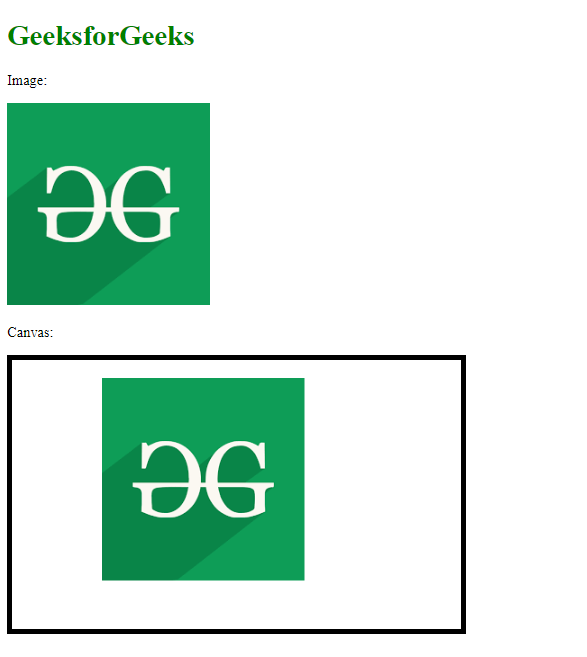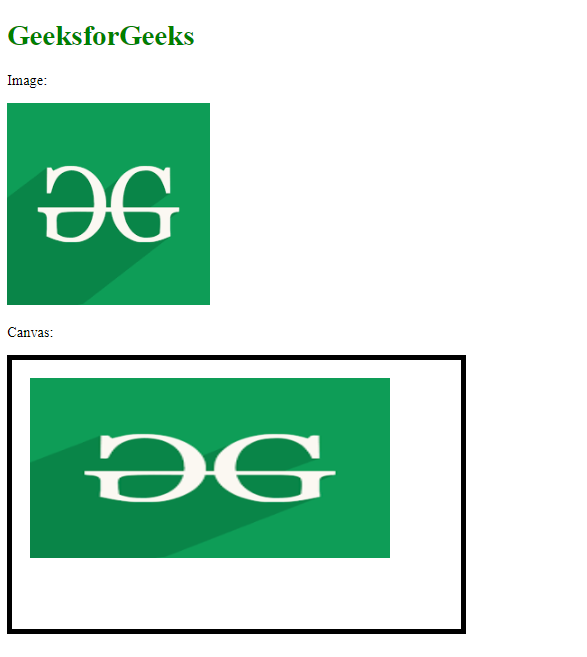Related Articles

# How to use the canvas drawImage() method in HTML5 ?

• Last Updated : 02 Sep, 2020

The canvas drawImage() method of the Canvas 2D API is used to draw an image in various ways on a canvas element. This method has additional parameters that can be used to display the image or a part of the image.

Syntax:

```context.drawImage(img, x, y, swidth, sheight, sx, sy, width, height);
```

Approach:

• Add image using the <img> tag.
• Draw the canvas using <canvas> tag.
• Load the canvas and get the context.
• Select the image to be used,
• Draw the image along with additional optional parameters, if required.

Example 1: In this example, the position of the image in the canvas is set using additional parameters.

## html

 ```<``html``>`` ` `<``body``>``    ``<``h1` `style``=``"color: green;"``>``        ``GeeksforGeeks``    ```` ` `    ``<``p``>Image:`` ` `    ``<``img` `id``=``"gfg_image"` `src``=``"https://media.geeksforgeeks.org/wp-content/uploads/20200604000733/gfg199.png"` `/>`` ` `    ``<``p``>Canvas:`` ` `    ``<``canvas` `id``=``"myGFGCanvas"` `width``=``"500"` `height``=``"300"` `        ``style``=``"border: 5px solid black"``>``    ````     ` `    ``<``script``>``        ``window.onload = function () {`` ` `            ``// Get the canvas element from the page``            ``var canvas = document.getElementById("myGFGCanvas");`` ` `            ``// Get the 2D context of the canvas``            ``var ctx = canvas.getContext("2d");`` ` `            ``// Get the image to be drawn on the canvas``            ``var image = document.getElementById("gfg_image");`` ` `            ``// Draw the image using drawImage() function`` ` `            ``// The first parameter is the image to be drawn`` ` `            ``// The second and third parameter is the``            ``// x and y position of the image in the canvas``            ``ctx.drawImage(image, 100, 20);``        ``}; ``    `````` ` ``

Output:Example 2: In this example, the position and dimensions of the image is set using additional parameters.

## HTML

 ```<``html``>`` ` `<``body``>``    ``<``h1` `style``=``"color: green;"``>``        ``GeeksforGeeks``    ```` ` `    ``<``p``>Image:`` ` `    ``<``img` `id``=``"gfg_image"` `src``=``"https://media.geeksforgeeks.org/wp-content/uploads/20200604000733/gfg199.png"` `/>`` ` `    ``<``p``>Canvas:`` ` `    ``<``canvas` `id``=``"myGFGCanvas"` `width``=``"500"` `height``=``"300"` `        ``style``=``"border: 5px solid black"``>``    ````     ` `    ``<``script``>``        ``window.onload = function () {`` ` `            ``// Get the canvas element from the page``            ``var canvas = document.getElementById("myGFGCanvas");`` ` `            ``// Get the 2D context of the canvas``            ``var ctx = canvas.getContext("2d");`` ` `            ``// Get the image to be drawn on the canvas``            ``var image = document.getElementById("gfg_image");`` ` `            ``// Draw the image using drawImage() function`` ` `            ``// The first parameter is the image to be drawn`` ` `            ``// The second and third parameter is the``            ``// x and y position of the image in the canvas`` ` `            ``// The fourth and fifth parameter is the``            ``// width and height of the image to be drawn``            ``// in the canvas``            ``ctx.drawImage(image, 20, 20, 400, 200);``        ``}; ``    `````` ` ``

Output:Attention reader! Don’t stop learning now. Get hold of all the important HTML concepts with the Web Design for Beginners | HTML  course.

My Personal Notes arrow_drop_up